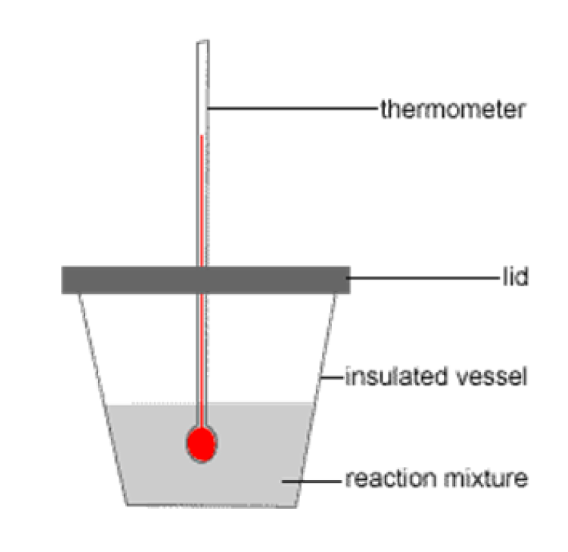# ΔH of Combustion and Neutralisation, Calorimetry

This is part of Year 11 HSC Chemistry course under the topic of Energy Changes in Chemical Reactions.

### HSC Chemistry Syllabus

• Conduct practical investigations to measure temperature changes in examples of endothermic and exothermic reactions, including:

– Combustion

• Investigate enthalpy changes in reactions using calorimetry and  𝑞 = 𝑚𝑐𝛥𝑇 (heat capacity formula) to calculate, analyse and compare experimental results with reliable secondary-sourced data, and to explain any differences

### ΔH of Combustion and Neutralisation, Calorimetry

This video will discuss how to calculate the enthalpy of Combustion and Neutralisation mathematically, as well as experimentally using a calorimetry device.

### Combustion Reactions

Combustion is a reaction that occurs between a combustible chemical and an oxidiser like oxygen. Combustion can either be complete or incomplete:

• Complete Combustion: produces carbon dioxide and water
• Incomplete Combustion: Yields a combination of carbon monoxide, soot, and carbon dioxide.

Example: The combustion of propane with oxygen, results in the formation of only carbon dioxide and water, indicating complete combustion.

$$C_3H_8(g) + 5O_2(g) \rightarrow 3CO_2(g) + 4H_2O(g)$$

### Acid-Base and Neutralisation Reactions

• Reactions between acids and bases involve the transfer of hydrogen ions H^+ from an acid to a base, typically resulting in the formation of water and a salt.

Example: Consider the reaction of hydrochloric acid (HCl) with sodium hydroxide (NaOH), leading to the formation of a salt (NaCl) and water

$$HCl(aq) + NaOH(aq) \rightarrow H_2O(l) + NaCl^–(aq)$$

### Energy Changes in Neutralisation:

• Neutralisation reactions are generally exothermic due to the formation of covalent bonds between H^+ and OH^– ions.
• The proticity of an acid, indicates the number of hydrogen ions it can donate, directly influences the reaction's exothermic nature

### Calorimetry

Calorimetry is an essential technique in chemistry and physics used to measure the amount of heat released or absorbed in a chemical reaction.

A calorimeter is an instrument which utilises calorimetry to measure heat changes.A calorimeter is designed to isolate a reaction or a substance and measure its heat transfer with surroundings. There are different types of calorimeters, each varying in complexity and precision, suited for specific types of measurements.

Types of Calorimeters:

1. Simple Calorimeters:
These are often used in educational settings and can be as basic as a coffee cup calorimeter. A coffee cup calorimeter consists of a polystyrene (Styrofoam) cup serving as an insulated container.
2. Bomb Calorimeters
More sophisticated and precise, bomb calorimeters are used in professional laboratories. They consist of a sealed container (the "bomb"), usually made of strong insulating metals where the reaction occurs in a controlled oxygen environment.

### Role of the q = mcΔT Equation

The equation q = mc \Delta T is central to the process of measuring heat changes in coffee cup calorimetry. It represents the relationship between heat energy (q), mass (m), specific heat capacity (c), and the change in temperature (\DeltaT).

• q: Heat energy absorbed or released in the reaction (J or kJ)
• m: Mass of the substance, often the solvent (g or Kg)
• c: Specific heat capacity, the amount of heat required to raise the temperature of 1 gram of a substance by 1 degree celsius (J g^{–1} °C^{–1}
• \DeltaT: Change in temperature observed during the reaction (in degrees celsius

Using the Coffee Cup CalorimeterFigure: Diagram of a coffee cup calorimeter. The styrofoam cup acts as an insulated vessel which keeps in heat produced by the reaction mixture

• Setup: A coffee cup calorimeter typically consists of a polystyrene (Styrofoam) cup, a lid, and a thermometer. The cup acts as an insulating vessel to minimise heat loss to the surroundings.

• Conducting the Experiment: The reactants are mixed in the cup, and the initial temperature is recorded. As the reaction proceeds, heat is absorbed or released, causing a change in the temperature of the solution.

• Applying the Equation: Once the final temperature is recorded, \Delta T is calculated as the difference between the final and initial temperatures. The mass (m) of the solution is measured, and the specific heat capacity (c) is generally assumed to be that of water (4.18 J g^{–1} °C^{–1}). Entering these values into the q = mc \Delta T equation calculates the total heat energy change.

Previous Section: Enthalpy Change, Activation Energy, Catalysts & Temperature

﻿Next Section: Enthalpy Change (ΔH) in Ionic Compound Dissolution